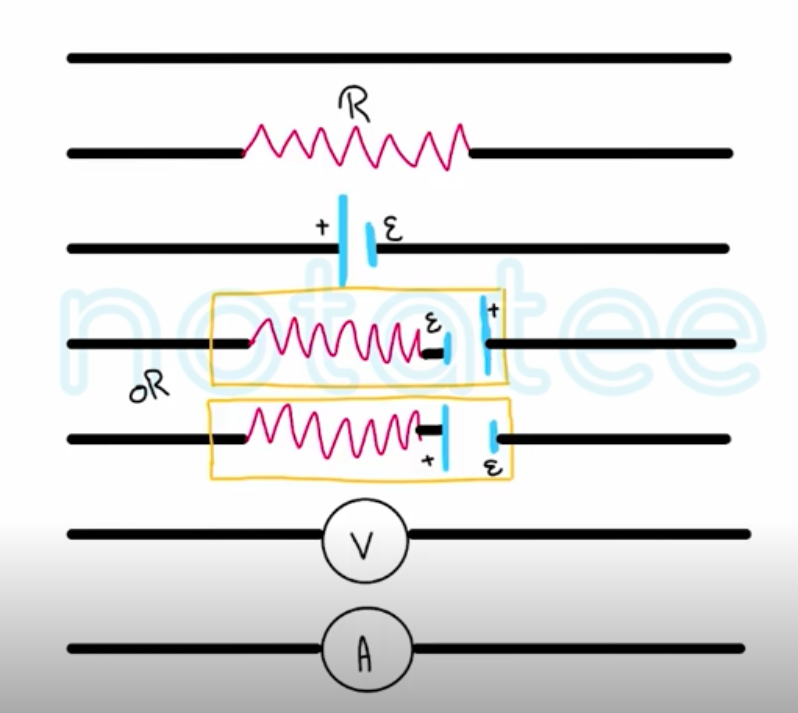Need Help?

Subscribe to Physics 2

###### \${selected_topic_name}
• Notes

* $\varepsilon=V_{a b}=I R$
$\text { (ideal source of emf) }$

* $V_{a b}=\varepsilon-I r$
$\text { (terminal Voltoge. }$$\text { Source with internal resistance) }$

* $\varepsilon-I r=I R$
OR        $I=\frac{\varepsilon}{R+r}$
$\text { (Current, Source with internal resistomce) }$